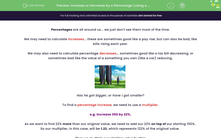# Increase or Decrease by a Percentage (using a multiplier)

In this worksheet, students will learn to increase or decrease an amount by using a multiplier, plus apply this skill to written problems.Key stage:  KS 4

Year:  GCSE

GCSE Subjects:   Maths

GCSE Boards:   AQA, Eduqas, Pearson Edexcel, OCR,

Difficulty level:#### Worksheet Overview

Percentages are all around us... we just don't see them most of the time.

We may need to calculate increases... these are sometimes good like a pay rise, but can also be bad, like bills rising each year.

We may also need to calculate percentage decreases... sometimes good like a tax bill decreasing, or sometimes bad like the value of a something you own (like a car) reducing.Has he got bigger, or have I got smaller?

To find a percentage increase, we need to use a multiplier.

e.g. Increase 350 by 22%.

As we want to find 22% more than our original value, we need to add our 22% on top of our starting 100%.

So our multiplier, in this case, will be 1.22, which represents 122% of the original value.

Then we multiply our starting value by this:

350 × 1.22 = 427

But what about if we are looking for a percentage decrease?

e.g. Decrease 350 by 22%.

You may be tempted to think that we need to complete the sum 350 × 0.22, right?

No, no, no! This tells us what 22% of 350 is, not how much remains after a 22% decrease

Care is needed here. Remember that 'percent' means out of 100

So we need to take the 22% from 100% first.

This makes sense if we give it some thought - we want to have 22% less than the value we started with, which represented 100%.

100 - 22 = 78

We only want to find the 78% that is left.

To complete the decrease, we calculate:

350 x 0.78 = 273Take it slowly and we will get there...

In this activity, we will calculate the percentage increases and decreases using raw numbers and in real-life situations.

You may want to have a calculator handy to use, so you can concentrate on practising these methods and not exhausting your mental maths brain!

Let's get started.

### What is EdPlace?

We're your National Curriculum aligned online education content provider helping each child succeed in English, maths and science from year 1 to GCSE. With an EdPlace account you’ll be able to track and measure progress, helping each child achieve their best. We build confidence and attainment by personalising each child’s learning at a level that suits them.

Get started